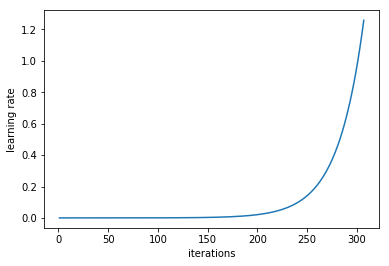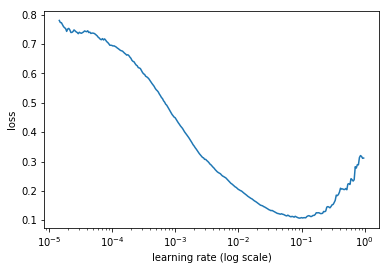# Lesson 2 discussion - beginner

what do u mean by regular password

@sree i am getting the same error “List index out of range” …What do u mean by regular password?

As in not use any other login credentials such as through Facebook/GitHub/LinkedIn etc. and use a regular user id/password combo.1 Like

@jeremy I want to verify my understanding about the working of the learning rate finder:From the above plot my understanding is :

• `Number of iterations = Number of rows/batch size (default = 64)` ~ 360 iterations. So in every iteration I’m applying SGD on mini batch of 64 images with a fixed learning rate and then in the next mini batch I increase the learning rate by some amount till I cover all the batches
• So for each mini batch using a constant learning rate SGD is implemented to get the minimum loss for that batch+LR combination and it’s plotted in the below plot
• Now if the above point is correct then why a small learning rate will give a high loss as shown in the graph as small learning rate will converge to minima (although it’ll take some time but it’ll, if we allow it to converge). Also the loss can be dependent on the kind of images in each batch.Please tell me if my understanding is right. Thanks!!

Yes that’s right, althought’s it’s kind of confusing to say “fixed learning rate” above, since for a particular learning rate we’re only doing a single mini-batch.

So the small learning rates are being used at the start of training, at a point when the loss is still high. Over time, the loss improves, and during that time the learning rate is also increasing. Hence the shape you see in the 2nd plot. Does that make sense?

I think the source of confusion it that you’re assuming we’re doing multiple SGD steps for each learning rate level, but we’re actually only doing one.

1 Like

Try this from @Jeremy:

`pip install git+https://github.com/floydwch/kaggle-cli.git`

My assumption is that let say we take a mini batch of 64 images and start with a learning rate of 1e-5. On this batch of 64 images I’ll do SGD once using LR = 1e-5 and store the minimum loss that I get. Now I pass another mini batch of 64 images but this time I increase the LR to some value let say 1e-4 and repeat the process. Is it how it is working ?

I’m not sure this is required any more - it looks like they’ve updated their software in pip. So just `pip install kaggle-cli` should suffice (or on Crestle, `pip3 install kaggle-cli`).

No that’s not what we’re doing - sorry that I didn’t explain this clearly enough. We just do a single step with that learning rate. So there’s no concept of a ‘minimum loss’ at the learning rate - there’s only one single mini batch, one single step, one loss.

So we:

1. Pick some initial LR
2. Grab one mini batch of images
3. Do a single step of SGD
4. Record the loss for that one mini batch after that one step
5. Increase the LR a little

Does that make more sense?..

4 Likes

Yeah now I got what is happening. But the loss that we are capturing after one step for a SGD can highly depend on where we are starting on the curve. If we start from somewhere very close to the minima then even a small LR can give us a low loss after one step. From one step of SGD I understand: Calculate the derivative at the current point and multiply by LR and subtract by the current value of weight to get the updated weight and then calculate the loss for that new weight. I guess I need to read about SGD.

That’s right. But we start at a random point in a space with millions of dimensions. So we’re definitely not going to be near the minimum point! That’s why the lr finder always starts with a high loss, and improves as we do more mini batches (until the lr gets too high).

Don’t worry if the reason why this works isn’t clear yet - for now the important things to understand are:

• What is it doing
• What do we use it for
• How do we use it in practice.

We’ll learn lots about SGD during the rest of the course.

@jeremy In the sequence of steps you have mentioned, it seems every succeeding learning rate is getting benefitted from every preceding one because every preceding one is adjusting the weights to move them closer to the minima (thereby reducing loss already).

That may not be fair to all the learning rates.

Should we be resetting the weights before starting a mini batch? (Or have the same starting point when a batch begins)

(Please ignore if you are going to cover this in later classes)

1 Like

Yeah I’m clear about the 3 points you mentioned. I guess subsequent classes will make it more clear. Thanks for the helpThat’s exactly right, and that’s why we’re not looking for the minimum point on the graph, but the point before that where the slope is clearly negative.

No that’s not what you want - you want to see how it improves during training. You would only want to reset the weights if you were looking for the minimum point in the plot, which isn’t what we’re looking for. Take a look at the last video where we discuss this.

2 Likes

Got it, look for the steepest decrease in loss. Thanks!

Nearly! The steepest point won’t work as well with SGDR. Something between that and the minimum point is likely to work best in practice. Try a couple of approaches and see what you find works best!

1 Like

I have some confusion regarding precompute parameter.
When precompute=True, we are using some pre-trained weights. How were these pre-trained weights initially obtained? Were they obtained by training on Imagenet images or on dog-cat images?
Another doubt is when learn.fit() is called just after creating learn object (where precompute=True), which weights does it update? Since precompute was set to True, weren’t all layers frozen?

Imagenet. Most are from here: http://pytorch.org/docs/master/torchvision/models.html

We’ll learn about this next week. But in short - we’re adding some new layers to the end of the pretrained model, and just updating them initially.

1 Like

One important point to mention: at this point, you’re not expected to have a strong intuition for why the techniques we’ve seen work; what’s important is that you understand in practice when and how to use them to train a good model.

So you should be spending as much time as possible writing and running code - initially using the datasets shown in class, and then using other datasets (e.g. kaggle dog breeds, or a dataset of your choice). Here’s some ideas on some basic things to try: http://wiki.fast.ai/index.php/How_to_use_the_Provided_Notebooks

We’ve found that students learn deep learning best when they spend as much time as possible actually using it; then when we dive more into the theory you’ll have a better understanding of how it fits in, in practice.

9 Likes

During learning with freeze, does our model traverse from input layer until output layer or the interaction is just between the 'last layer (from the model which has all activations) and the output layer(s)?

Confusion: When the state is frozen, we aren’t updating activations thus going back and forth doesn’t make sense but talking to the last layer which is sort of input layer for the layers which we’ve added.